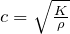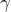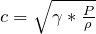# Speed of Sound in Gas

Speed of Sound in an Ideal Gas

Summary

The wave speed of a fluid is equivalent to the speed of sound in the same medium, whether liquid or gas.

The speed of sound is calculated from the Newton-Laplace equation:where c = speed of sound, K = bulk modulus or stiffness coefficient, ρ = density.

For an ideal gas, the bulk modulus K =* P where(gamma) = adiabatic index or isentropic expansion factor, P = pressure.

The isentropic expansion factor is the ratio of specific heats of a gas at constant pressure and constant volume (i.e. Cp / Cv). For air, this ratio is approximately 1.4 when assumed to be ideal and at 0°C.

Therefore for an ideal gas, the wave speed is given as:Speed of Sound in an Ideal Gas Calculator

 Calculate speed of sound in gas using isentropic expansion factor (ratio of specific heats): Formula:c = speed of sound= isentropic expansion factor (Cp / Cv). (Approx 1.4 for air) ρ = density Isentropic expansion factor Density kg/m³ Pressure Pa Result: m/s